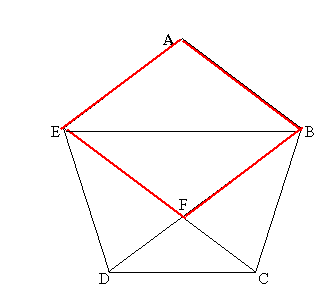#### You may also like### Golden Thoughts

Rectangle PQRS has X and Y on the edges. Triangles PQY, YRX and XSP have equal areas. Prove X and Y divide the sides of PQRS in the golden ratio.### At a Glance

The area of a regular pentagon looks about twice as a big as the pentangle star drawn within it. Is it?### Areas and Ratios

Do you have enough information to work out the area of the shaded quadrilateral?

# Pent

##### Age 14 to 18Challenge Level

There are a few points worth thinking about when you tackle a problem like this:

• When the solution is given in surds this is really a signal not to use trigonometric tables or a calculator. One of the problems with using calculators and tables is that you will calculate lengths and angles to a certain degree of accuracy (say 10 decimal places) but even if two numbers are equal to 10 decimal places, this does not mean that they are equal.
• When you are tempted to use trigonometry and you are not using angles whose trigonometric ratios can be written easily as surds, remember that trigonometry is based on the properties of similar triangles. It is often the case that the most elegant solution in these situations involves the direct use of similar triangles.
• It is enough that the four sides of a quadrilateral are equal to be able to say it is a rhombus (why?).
• When you write proofs, try to structure them so that you make it easy for the reader to follow. Think of "chunking" your proofs so that each part stands on its own as much as possible.

Jacqui Eaves made a good attempt at this problem and Andrei Lazanu's work forms the basis of the solution below, well done Andrei.

The solutionFirst I calculated the angle between two sides of the pentagon.
I used the formula for the sum of angles of a regular polygon, with n sides, that is:

180 °(n-2)

For the pentagon, I obtained: $${180^{\circ}(n - 2)} = {180^{\circ}(5 - 2)} = {180^{\circ}\times 3} = {540^{\circ}}$$ Therefore the angle in each vertex is ${540^{\circ}\over5} = {108^{\circ}}$ $${\angle EAB}\equiv{\angle ABC}\equiv{\angle BCD}\equiv{\angle CDE}\equiv{\angle DEA} = {108^{\circ}}$$

Triangles CDE, DCB and AEB are isosceles (sides of pentagon are one unit) $${\angle DEC}\equiv{\angle DCE}\equiv{\angle BDC}\equiv {\angle CBD}\equiv{\angle AEB}\equiv{\angle ABE} = {{180^{\circ} - 108^{\circ}}\over2} = {72^{\circ}\over2} = {36^{\circ}}$$

Then I calculated other sets of equal angles: $${\angle DFC}\equiv{\angle EFB}= {180^{\circ} - 2\times 36^{\circ}} = {108^{\circ}}$$ $${\angle EFD}\equiv{\angle BFC}= {{360^{\circ} - 2\times 108^{\circ}}\over2} = {{360^{\circ} - 216^{\circ}}\over2} ={144^{\circ}\over2}= {72^{\circ}}$$ $${\angle EDF}\equiv{\angle BCF}= { 180^{\circ} - 36^{\circ} - 72^{\circ}} = { 72^{\circ}}$$ $${\angle BEF}\equiv{\angle EBF}= {{180^{\circ} - 108^{\circ}}\over2} = {72^{\circ}\over2} = {36^{\circ}}$$

Here are all the measures of the angles that I obtained:

 Angles Measures ${\angle EAB}\equiv{\angle ABC}\equiv{\angle BCD}\equiv{\angle CDE}\equiv{\angle DEA}$ 108 ° ${\angle EAB}\equiv{\angle ABC}\equiv{\angle BCD}\equiv{\angle CDE}\equiv{\angle DEA}$ 36 ° ${\angle DFC}\equiv{\angle EFB}$ 108 ° ${\angle EFD}\equiv{\angle BFC}$ 72 ° ${\angle EDF}\equiv{\angle BCF}$ 72 ° ${\angle BEF}\equiv{\angle EBF}$ 36 °
The four sided polygon AEFB is a rhombus because it has all sides equal (one unit)

Triangles EFB and DFC are both isosceles, their corresponding angles are equal

I used the following notation:

• DF = x
• FB = 1 (equal in length to the side of the pentagon)
• EB = r

Using the relationship obtained using the similarity of the triangles, I obtain: $${x\over1} = {1\over{r}}$$

I know that r = x + 1 because triangle BED is also isosceles.
x and r are the solutions of the following system of two equations: $${x\over1} = {1\over{r}}$$ $${x + 1} = {r}$$

I calculate the two numbers, x and r , substituting r from the second equation into the first. I obtain an equation of the form: $ax^2 + bx + c = 0$ Which has the solutions: $${x_1,x_2 }={-b\pm{\sqrt{{b^2}-4ac}}\over{2a}}$$

Solving the equation obtained for x, I obtain successively: $${x(x+1)} = {1}$$ $${x^2 + x} = {1}$$ $${x^2 + x - 1} = {0}$$ $${a = 1}, {b = 1} , {c = -1}$$ $${x_1} = {-1 + {\sqrt{1+4}}\over2} = {{-1 + \sqrt5}\over2} = {{ 1\over{2}} (\sqrt{5} - 1)}$$ $${x_2} = {{-1\sqrt5}\over2}$$

The last solution is not a solution for the problem, because x cannot be negative, but the first one is. So I proved that $${x } = {{ 1\over{2}} (\sqrt{5} - 1)}$$

Now I calculate r, that is x + 1. $${r} = {x + 1} = {{{\sqrt{5} - 1}\over2} + 1} = { {\sqrt{5} + 1}\over2}$$So the length of the chord EB of the pentagon is equal to the golden ratio $(\sqrt 5 + 1)/2$.

In conclusion: $${x } = {{ 1\over{2}} (\sqrt{5} - 1)}.$$ The ratio BF : FD is equal to $1/x$ so this ratio is $${2\over {\sqrt 5 -1}} ={ 2({\sqrt 5 + 1}) \over (\sqrt 5 - 1)( \sqrt 5 + 1)}= {\sqrt 5 + 1 \over 2}.$$ So F divides the chord BD in the golden ratio.